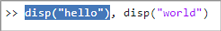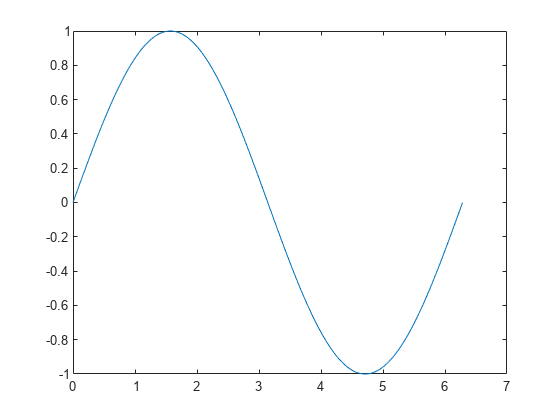# 命令行窗口

## 说明## 示例

`a = 1`
```a = 1 ```

`sin(a)`
```ans = 0.8415 ```

`b = a + 2;`

`A = magic(5), B = ones(5) * 4.7; C = A./B`
```A = 17 24 1 8 15 23 5 7 14 16 4 6 13 20 22 10 12 19 21 3 11 18 25 2 9 C = 3.6170 5.1064 0.2128 1.7021 3.1915 4.8936 1.0638 1.4894 2.9787 3.4043 0.8511 1.2766 2.7660 4.2553 4.6809 2.1277 2.5532 4.0426 4.4681 0.6383 2.3404 3.8298 5.3191 0.4255 1.9149```

```x = 0:pi/100:2*pi; y = sin(x); plot(x,y)````commandwindow`

## 编程用途

`commandwindow` 选择 MATLAB 命令行窗口。例如，在脚本或实时脚本中，在绘图命令后键入 `commandwindow` 以使焦点返回到命令行窗口。

## 提示

• 要根据命令行窗口的当前大小来确定其中显示的列数和行数，可以在命令行窗口中键入 `matlab.desktop.commandwindow.size`。MATLAB 分别返回列数和行数。例如：

`matlab.desktop.commandwindow.size`
```ans = 133 24```

如果选择将矩阵显示宽度设置为 80 列命令行窗口预设项，则列数为 80。有关详细信息，请参阅设置命令行窗口预设项

• 要在命令行窗口中显示文本或变量值，可以使用 `disp` 函数。例如，以下代码创建一个变量，并在命令行窗口中显示其值。

```A = [15 150]; disp(A)```
` 15 150`
您也可以使用 `fprintf` 函数来显示文本。